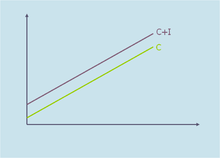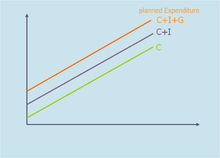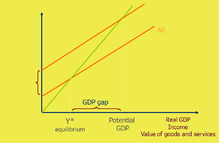# Aggregate expenditure

In economics, aggregate expenditure (AE) is a measure of national income. Aggregate expenditure is defined as the current value of all the finished goods and services in the economy. The aggregate expenditure is thus the sum total of all the expenditures undertaken in the economy by the factors during a given time period. It refers to the expenditure incurred on consumer goods, planned investment and the expenditure made by the government in the economy. In an open economy scenario, the aggregate expenditure also includes the difference between the exports and the imports.

Aggregate expenditures is defined as : AE = C+Ip+G+NX,

• C = Household Consumption
• Ip = Planned Investment
• G = Government spending
• NX = Net exports (Exports-Imports)

Aggregate expenditure is one of the methods to calculate the sum total of all economic activities in an economy, which is referred to as the gross domestic product of an economy. The gross domestic product which is an important measure of the growth of the economy is calculated through the aggregate expenditure model, also known as the Keynesian cross. AE is also used in the aggregate demand-aggregate supply model which advances the aggregate expenditures model with the inclusion of price changes.

Components of aggregate expenditure (AE) – defined as the total amount that firms and households plan to spend on goods and services at each level of income. Also, it can be seen that the aggregate expenditure is the sum of expenditures on consumption, investment, government expenses and net exports. It is normally derived from all the components of the aggregate demand. Aggregate demand (AD) refers to the sum total of goods that are demanded in an economy over a period and thus AD is defined by the planned total expenditure in an economy for a given price level.

## Components

Various schools of thoughts use various components to come up with the Aggregate Expenditure. The major school of economic thoughts which are the classical and Keynesian economists use the following components:

• Consumption expenditure (C)

Consumption refers to the household consumption over a given period of time. The total household consumption can be divided into two parts, they are: Autonomous Consumption and Induced consumption. Autonomous Consumption refers to the amount of consumption regardless of the amount of income, hence, even if the income is zero, the autonomous consumption would be the total consumption. Induced consumption refers to the level of consumption dependent on the level of income.

• Y = Income
• Investment (I)

Investment is the amount of expenditure towards the capital goods. Investment refers to the expenditure towards goods that are expected to yield a return or increase their own value over time. The investment expenditure can be further divided into two parts, planned investment and unplanned investment. Over the long run the sum of differences in the unplanned investment would equal to zero as economy approaches equilibrium.

• Government Expenditure:(G)

The Keynesian model propagates an active state to control and regulate the economy. The government can make expenditure in terms of infrastructure, thus increase the total expenditure in the economy as advocated by Keynes. Transfer payments (such as pensions and unemployment benefits) are NOT included in G as that would mean a double count.

• Net Exports:(NX)

In an open economy, the total expenditure of the economy also includes the components of the net exports which is the total exports minus the total imports.

## Classical economics

Classical economists relied on the Say's law which states that supply creates its own demand, which stemmed from the belief that wages,prices and interest rates are all flexible. This comes from the classical thought that the factor payments which are made to the various factors of production during the production process, would create enough income in the economy to create a demand for the products produced.Classical Aggregate Expenditure

This supports the classical thought which revolves around Adam Smith's invisible hand which states that the markets would achieve equilibrium via the market forces that impact economic activity and thus there is no need for government intervention. Moreover, the classical economists believed that the economy was operating at a full employment Hence the classical Aggregate expenditure model is:

Aggregate Expenditure = Aggregate Consumption + Planned Investment

Therefore,Where,

• C= Consumption Expenditure
• I = Aggregate investment ( Savings = Investment)

Classical economics has been criticized for its assumptions that the economy works on a full-employment equilibrium which is a false assumption in reality, where the economy often operates at an under-employment equilibrium mainly because of "sticky" wages, which in turn provides the foundation for the Keynesian model of aggregate expenditure.

## Keynesian economics

Keynesian economics believes, contrary to the classical thought that the wages, prices and interest rates are not flexible and hence violating Say's law, which provided the foundation for the maxim that "supply creates its own demand". Keynes believed that the economy was subject to sticky prices and thus the economy was not in a state of perpetual equilibrium and also operated at an under-employment equilibrium.Keynesian aggregate expenditure

Keynesian economics calls for a government intervention and is called demand side economics as it believes that aggregate demand and not the aggregate supply determines the GDP because of the difference between the aggregate supply and planned expenditure in an economy. Hence Keynes believed that the government played an important role in the determination on the aggregate expenditure in an economy and was thus included government expenditure in the aggregate expenditure function.

Hence,

•Where,

• C = Household Consumption Expenditure
• I = Investment (Planned)
• G = Government Expenditure
• NX= Net Exports (Exports − Imports)

Keynesian economics preaches that in times of a recession, the government must undertake the expenditure to compensate for the lack in the components of Household expenditure (C) and private investment (I) so as to ensure that the demand is maintained in the markets. This also leads to the Keynesian multiplier which suggests that every dollar spent on investment creates a multiplier effect and leads to an increased expenditure of more than one dollar.

## Aggregate supplyeffect of increase in expenditure

An economy is said to be in equilibrium when aggregate expenditure is equal to the aggregate supply (production) in the economy. According to Keynes, the economy does not stay in a perpetual state of equilibrium but the Aggregate expenditure and Aggregate Supply adjust each other towards equilibrium. When there is an excess supply over the expenditure and hence the demand there is an inventory leftover with the producers, which leads to a reduction in either the prices or the quantity of output and hence reducing the total output (GDP) of the economy. On the other hand, if there is an excess of expenditure over supply, then there is excess demand leading to an increase in prices or output. Hence the economy constantly keeps shifting between excess supply (inventory) and excess demand. Thus, the economy is constantly moving towards an equilibrium between the aggregate expenditure and aggregate supply. In an under-employment equilibrium the Keynesian cross refers to the point of intersection of the aggregate supply and the aggregate expenditure curve. The rise in the expenditure by either Consumption (C), Investment (I) or the Government (G) or an increase in the exports or a decrease in the imports leads to a rise in the aggregate expenditure and thus pushes the economy towards a higher equilibrium and thus reaching a higher level towards the potential of the GDP.

## References

1. Trosten, Jochem (2009). Macro-recitation (PDF). pp. 2–7.
2. Haworth, Barry. "The Aggregate Expenditure Model". The Aggregate expenditure model. University of Louisville. Retrieved 13 November 2011.
3. Rittenberg, Tregarthen, Libby,Timothy. Principles of Macroeconomics.
4. "Components of Aggregate Expenditure" (PDF). Stirling University. Retrieved 13 November 2011.
5. Byrns. Student Guide for Learning Contemporary Economics (PDF). p. 127.
6. Burnette, Jeffery. Classical Economics- Principles of Micro (PDF). Rochester Institute of Technology. p. 9.
7. Burnette, Jeffery. Classical Economics- Principles of Micro (PDF). Rochester Institute of Technology. p. 9.
8. Branson, William (1979). Macroeconomic theory and policy.
9. "Aggregate Expenditure" (PDF). Keynesian Model. Retrieved 13 November 2011.
• Parry G., and Kemp S., (2009) Discovering Economics Tactic Publications, South Perth.
This article is issued from Wikipedia - version of the 11/27/2016. The text is available under the Creative Commons Attribution/Share Alike but additional terms may apply for the media files.